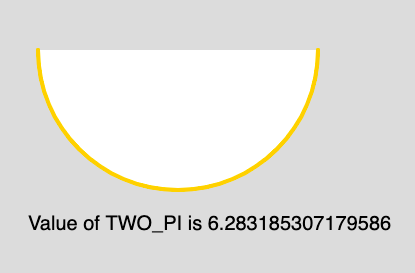GeeksforGeeks App
Open AppBrowser
Continue

## Related Articles

The TWO_PI is a mathematical constant with the value 6.28318530717958647693. It is twice the ratio of the circumference of a circle to its diameter.

Syntax:

`TWO_PI`

Below program illustrates the usage of TWO_PI in p5.js:

Example:

 `function` `setup() {``     ` `    ``// Create Canvas of size 880*300  ``    ``createCanvas(880, 300);``}``  ` `function` `draw() {``  ` `    ``// Set the Background Color``    ``background(220);``  ` `    ``// Set the stroke color``    ``stroke(255, 204, 0);``  ` `    ``// Set the stroke weight``    ``strokeWeight(4);``  ` `    ``// Draw Arc``    ``// Use of constant TWO_PI``    ``arc(180, 50, 280, 280, 0, TWO_PI/2);``  ` `    ``// Unset the stroke``    ``noStroke();``  ` `    ``// Set the text size``    ``textSize(20);``     ` `    ``// Display output``    ``text(``"Value of TWO_PI is "``+ TWO_PI, 30, 230);``}`

Output:Reference: https://p5js.org/reference/#/p5/TWO_PI

My Personal Notes arrow_drop_up
Related Tutorials